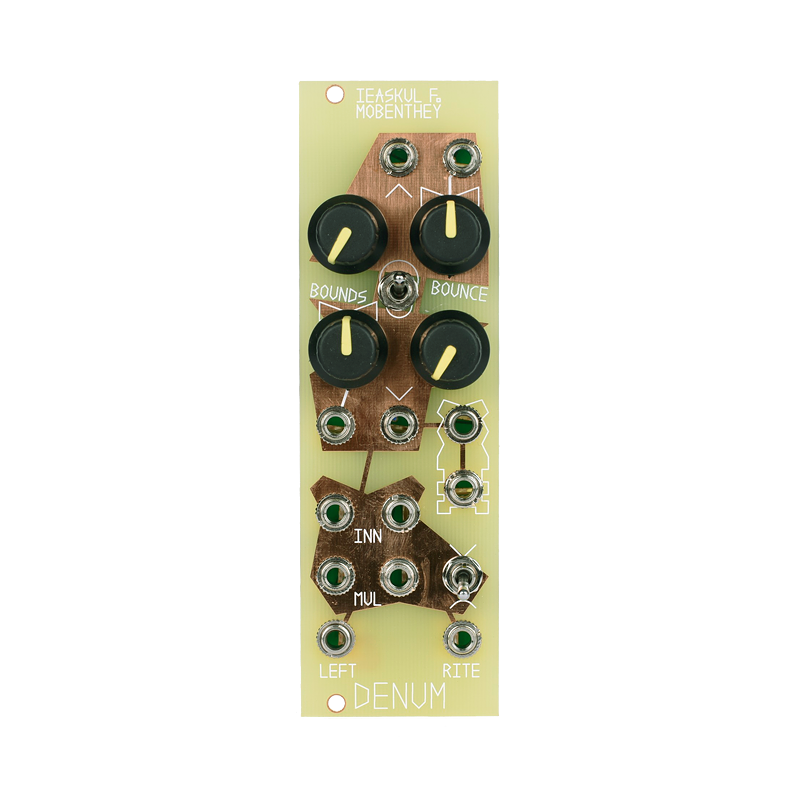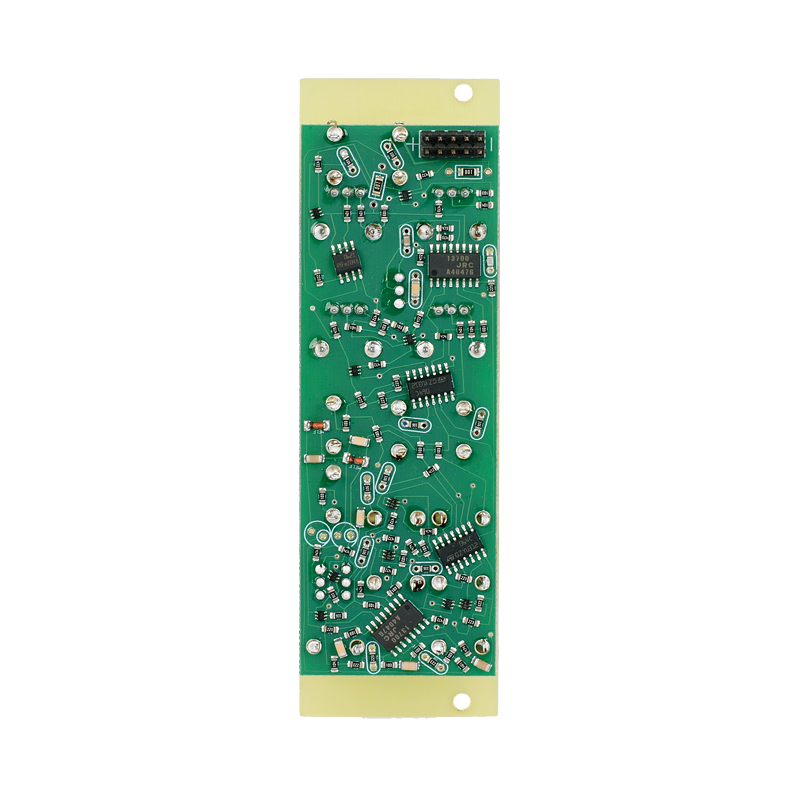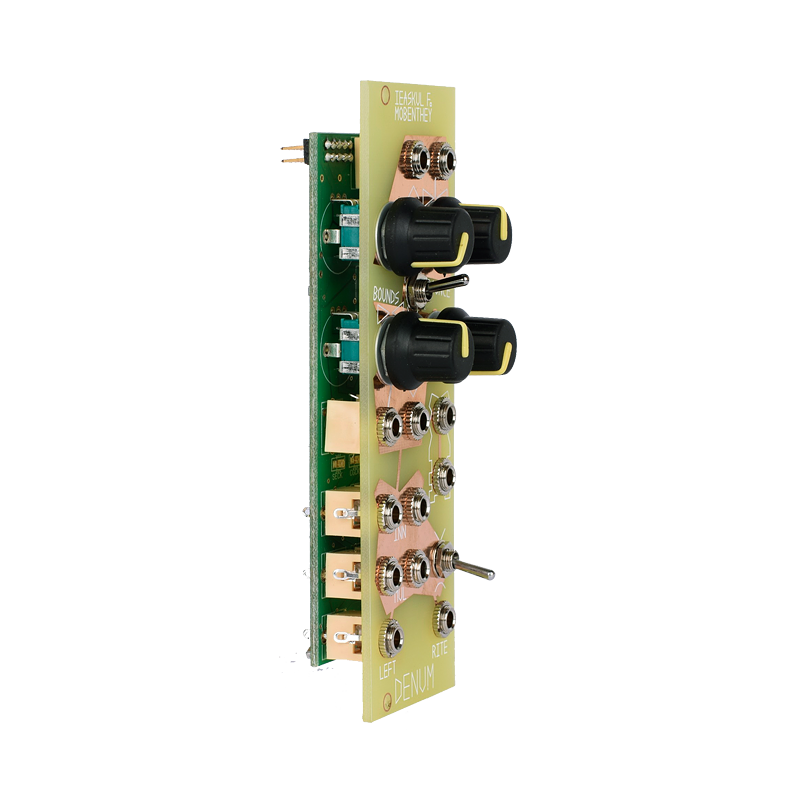Write a review
Regular price
\$222.00
Sale price
\$222.00
Regular price

/ /
The Denum module is essentially a bounds/bounce oscillator. It has the “triangle core” circuitry used in many oscillators, but it adds a correlated bounds modulation, according to the concept (see below). Here, bounds and bounce are each given equal treatment, with separate linear and exponential controls and inputs. In addition, this module has a fully featured bi-polar VCA with complimentary inputs, to get you quickly going sending “bb-objets” out the left and right channels.

Denum is an 8HP Eurorack module that runs on +12 and -12 volts. Attach power connector positive to “+” and negative to “-”. Failure to follow proper power polarity will result in instant destruction of unit.

Looking at the front panel of Denum, note that inputs are marked by copper fill. There are three main sections: bounce, bounds, and the VCA. Bounce is above bounds to emphasize the numerator/denominator relationship of the two. Each of the two has exactly the same inputs and knobs. The “basis” knob sets the base rate or position for bounds. There is a linear control input, marked by a a caret. Linear modulation is an alternative input for pre-enveloped audio, since it has no attenuverter. However, the exponential control input is tied to an attenuverter, marked by a characteristic bow-tie. An attenuverter works like this: at noon the modulations are nulled out, they have no effect; clockwise from there they increase in intensity, with positive input meaning “more”; to the counter-clockwise direction, modulations increase as well, but with negative input meaning “more”. This knob is essential to controlling how much, and in which direction, your modulations apply.

Between the bounds and bounce areas, there is a range switch. When in middle position, Denum runs at a standard audio rate. Pointing downward is a low audio rate, and pointing upwards is a definite CV, lowest rate.

The triangle output speaks the position of the waveform in voltage, and square expresses the frequency in terms of negative and positive 10 volts.

The triangle output is bussed into the left and right audio inputs of the VCA, at bottom. Either can be swapped out for external audio energies, via the “left, right signal inserts”. The “left, right VCA inputs” are for controlling the amplitude of the signals, with either a linear or exponential mapping. If the left input is greater than right, sound goes out the “left out”. If right is greater than left, sound goes out the “right out”. The voltage amount of difference maps to loudness in these channels. The “linear, expo switch” chooses linear when cocked up, as marked by a caret, and exponential when cocked down, as marked by a rounded form. There is an optional mute when the switch is in the middle.
The Denum module is essentially a bounds/bounce oscillator. It has the “triangle core” circuitry used in many oscillators, but it adds a correlated bounds modulation, according to the concept (see below). Here, bounds and bounce are each given equal treatment, with separate linear and exponential controls and inputs. In addition, this module has a fully featured bi-polar VCA with complimentary inputs, to get you quickly going sending “bb-objets” out the left and right channels.

Denum is an 8HP Eurorack module that runs on +12 and -12 volts. Attach power connector positive to “+” and negative to “-”. Failure to follow proper power polarity will result in instant destruction of unit.

Looking at the front panel of Denum, note that inputs are marked by copper fill. There are three main sections: bounce, bounds, and the VCA. Bounce is above bounds to emphasize the numerator/denominator relationship of the two. Each of the two has exactly the same inputs and knobs. The “basis” knob sets the base rate or position for bounds. There is a linear control input, marked by a a caret. Linear modulation is an alternative input for pre-enveloped audio, since it has no attenuverter. However, the exponential control input is tied to an attenuverter, marked by a characteristic bow-tie. An attenuverter works like this: at noon the modulations are nulled out, they have no effect; clockwise from there they increase in intensity, with positive input meaning “more”; to the counter-clockwise direction, modulations increase as well, but with negative input meaning “more”. This knob is essential to controlling how much, and in which direction, your modulations apply.

Between the bounds and bounce areas, there is a range switch. When in middle position, Denum runs at a standard audio rate. Pointing downward is a low audio rate, and pointing upwards is a definite CV, lowest rate.

The triangle output speaks the position of the waveform in voltage, and square expresses the frequency in terms of negative and positive 10 volts.

The triangle output is bussed into the left and right audio inputs of the VCA, at bottom. Either can be swapped out for external audio energies, via the “left, right signal inserts”. The “left, right VCA inputs” are for controlling the amplitude of the signals, with either a linear or exponential mapping. If the left input is greater than right, sound goes out the “left out”. If right is greater than left, sound goes out the “right out”. The voltage amount of difference maps to loudness in these channels. The “linear, expo switch” chooses linear when cocked up, as marked by a caret, and exponential when cocked down, as marked by a rounded form. There is an optional mute when the switch is in the middle.
The Denum module is essentially a bounds/bounce oscillator. It has the “triangle core” circuitry used in many oscillators, but it adds a correlated bounds modulation, according to the concept (see...

## Questions

Have any questions? We're here to help!